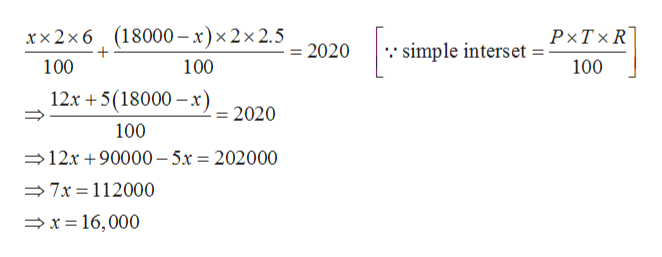Jenny borrowed a total of \$18.000 from two different banks to start a business. One bank charged the equivalent of 6% simple interest, and the other charged 2.5% simple interest. If the total interest after 2 years was \$2020, determine the amount borrowed from each bank.Jenny borrowed \$x at 6%?Jenny borrowed \$Y at 2.5%?

Question

Jenny borrowed a total of \$18.000 from two different banks to start a business. One bank charged the equivalent of 6% simple interest, and the other charged 2.5% simple interest. If the total interest after 2 years was \$2020, determine the amount borrowed from each bank.

Jenny borrowed \$x at 6%?

Jenny borrowed \$Y at 2.5%?

Step 1

Given,

Jenny borrowed a total of \$18.000 from two different banks to start a business. One bank charged the equivalent of 6% simple interest, and the other charged 2.5% simple interest and the total interest after 2 years was \$2020.

Step 2

Let jenny borrowed \$x from the first bank.

Then he must borrow \$(18000 - x) from the other bank, because total amount borrowed by him is \$18,000.

Step 3

Then,

...help_outlineImage Transcriptionclose"simple interset P-TXR 100 xx2x6 (18000- x)x2x 2.5 0 + 100 100 12x 5(18000 x 2020 100 12x 90000-5x = 202000 7x 112000 x16,000 fullscreen

Want to see the full answer?

See Solution

Want to see this answer and more?

Our solutions are written by experts, many with advanced degrees, and available 24/7

See Solution
Tagged in

Other